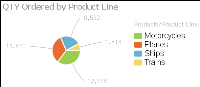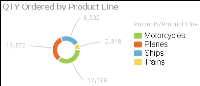Working with pie charts
A pie chart is a circular chart that is divided into sectors or slices. Each sector represents a value that is proportional to the sum of the values. Use a pie chart to show the relationship of parts to the whole, for example, the order quantity each product line contributes to the total, as shown in Figure 9‑6.Figure 9‑6 A pie chart
Working with doughnut charts
Doughnut charts are similar to pie charts. Doughnut charts are ring charts that consist of segments. Each segment represents a value that is proportional to the total value, as shown in Figure 9‑7.Figure 9‑7 A doughnut chart